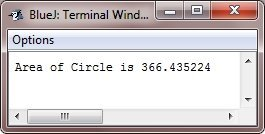# Java Floating Point Types

Floating-point or real numbers are used when evaluating the expressions that require fractional precision. For instance, calculations like square root, or transcendentals such as sine and cosine, result in a value whose precision requires the floating-point type.

Java implements the standard (IEEE-754) set of floating-point types and the operators. There are two kinds of floating-point types that is, float and double, which represent single-precision and double-precision numbers, respectively.

### Floating-Point Data Types Width and Range

The following table shows the width and range of the floating-point types :

Name Width in Bits Approximate Range
double 64 4.9e-324 to 1.8e+308
float 32 1.4e-045 to 3.4e+038

Now, let's take a look at these (double and float) floating-point types one by one.

### float

The type float specifies a single-precision value that uses 32-bits of storage. Single precision is more faster on some processors and takes half as much space as double precision, but will become inaccurate when the values are either very large or very small.

Variables of the type float are useful when you need a fractional component, but do not require a large degree of precision. For instance, float can be useful whenever representing the dollars and cents.

Below are some example of float variable declarations :

`float hightemp, lowtemp, rad;`

### double

Double precision is denoted by the keyword double, which uses 64-bits to store a value.

Double precision is actually faster than single precision on several modern processors that have been optimized for the high-speed mathematical calculations. All transcendental math functions, like sin(), cos(), and sqrt(), return double values. When you need to maintain the accuracy over many repetitive calculations, or are manipulating large-valued numbers, double is the best choice.

Following is a short and simple program which uses the double variables to compute the area of a circle :

```/* Java Program Example - Java Floating-Point Types
* Compute the area of a Circle
*/

public class JavaProgram
{
public static void main(String args[])
{

double pi, r, a;

r = 10.8;     // radius of the circle
pi = 3.1416;   // approximate value of pi
a = pi * r * r;     // compute area of the circle

System.out.println("Area of Circle is " +a);

}
}```

When the above Java program is compile and run, it will produce the following output:## Examples

Here are the list of some example programs that uses float and double (floating-point) type variables:

Tools
Calculator